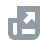Pay with
Markets
NFT
New
English
USDAccount FunctionsTutorialBinance Fan TokenBinance EarnCrypto Deposit/WithdrawalCrypto DerivativesFinanceAPISecurityNFTVIP

# Daily Interest Rate for Binance Margin Trading

2019-07-05 05:38
Binance’s margin account interest rate is calculated on an hourly basis.
NOTE: If funds are borrowed for less than 1 hour, the interest rate will still be calculated as for assets borrowed for 1 hour.
If the daily interest rate is 0.02%, the hourly interest rate is calculated as 0.02%/24.
The calculation formula: I (interest) = P (borrowed money) * R (daily interest 0.02%/24) * T (in hours)
For example:
If user A borrows 1,000 USDT at 13:20 PM, and repays at 14:15 PM, the interest rate is calculated as 1,000 *（0.02%/24）* 2 = 0.01666667 USDT.
Margin account interest rates may be adjusted from time to time. Please refer here to see the latest interest rates, borrow limits and other details regarding Margin trading.